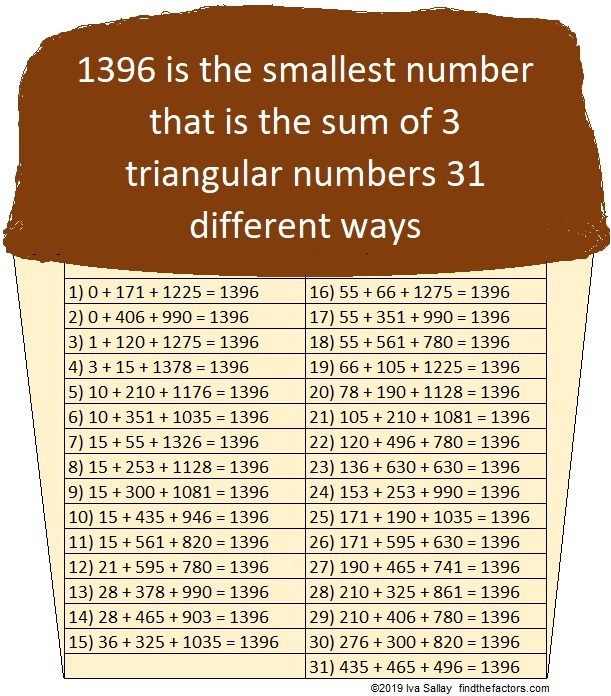# 31 Flavors of 1396

The first 52 triangular numbers are 0, 1, 3, 6, 10, 15, 21, 28, 36, 45, 55, 66, 78, 91, 105, 120, 136, 153, 171, 190, 210, 231, 253, 276, 300, 325, 351, 378, 406, 435, 465, 496, 528, 561, 595, 630, 666, 703, 741, 780, 820, 861, 903, 946, 990, 1035, 1081, 1128, 1176, 1225, 1275, 1326, 1378.

OEIS.org informs us that 1396 can be written as the sum of three triangular numbers in 31 different ways. It is the smallest number that can make that claim!That 31st way is written with three consecutive triangular numbers, 435, 465, and 496, which are the 29th, 30th, and 31st triangular numbers respectively. That fact makes 1396 the 31st Centered Triangular Number as well!That is, at least, 1396 is the 31st number on the list. You can also calculate it using this formula: [3(30²) + 3(30) + 2]/2 = 1396

Here’s more about the number 1396:

• 1396 is a composite number.
• Prime factorization: 1396 = 2 × 2 × 349, which can be written 1396 = 2² × 349
• 1396 has at least one exponent greater than 1 in its prime factorization so √1396 can be simplified. Taking the factor pair from the factor pair table below with the largest square number factor, we get √1396 = (√4)(√349) = 2√349
• The exponents in the prime factorization are 2 and 1. Adding one to each exponent and multiplying we get (2 + 1)(1 + 1) = 3 × 2 = 6. Therefore 1396 has exactly 6 factors.
• The factors of 1396 are outlined with their factor pair partners in the graphic below.1396 is the sum of two squares:
36² + 10² = 1396

1396 is the hypotenuse of a Pythagorean triple:
720-1196-1396 calculated from 2(36)(10), 36² – 10², 36² + 10²

This site uses Akismet to reduce spam. Learn how your comment data is processed.